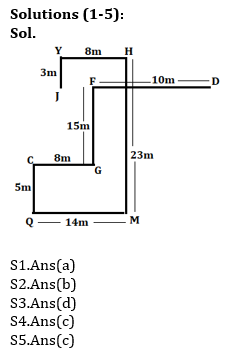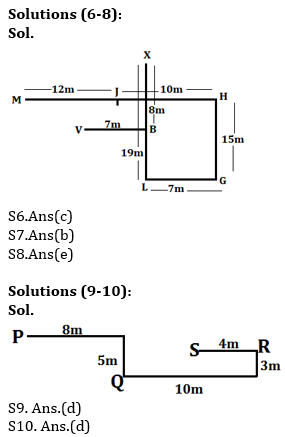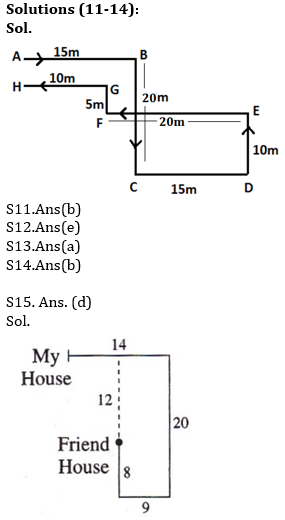Latest Banking jobs   »   Reasoning Ability Quiz For IBPS Clerk...

# Reasoning Ability Quiz For IBPS Clerk Prelims 2021- 27th July

Directions (1-5): Study the information carefully and answer the questions given below.
Adarsh starts his journey from point D, walks 10m towards west to reach at point F, then takes a left turn and walks for 15m to reach point G. From point G he takes a right turn and walks for 8m to reach at point C, From point C he takes a left turn and walks 5m to reach at point Q, then takes a left turn again and walks 14m to reach point M. From point M, He walks 23m in north direction and reached point H. From point H, he takes left turn and walks 8m to reach at point Y. From point Y, he takes left turn and walks 3m to reach point J.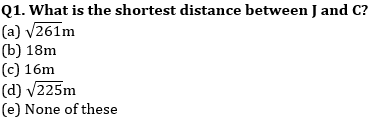Q2. In which direction point D with respect to point J?
(a) West
(b) East
(c) North-west
(d) South-east
(e) None of these

Q3. In which direction point F with respect to M?
(a) North
(b) South
(c) South-east
(d) North-west
(e) None of these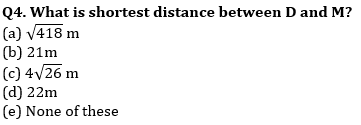Q5. If point N is 6m north of Point C, then what is the shortest distance between G and N?
(a) 7m
(b) 8m
(c) 10m
(d) 4m
(e) None of these

Directions (6-8): Study the information carefully and answer the questions given below.
Point J is 12m east of point M. Point B is 7m east of Point V. Point J is 10m west of Point H. Point L is 19m south of Point X. Point H is 15m north of Point G which is 7m east of Point L. Point X is 8m north of Point B.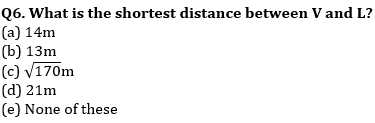Q7. Point V is in which direction with respect to H?
(a) North
(b) South-West
(c) South-East
(d) North-West
(e) West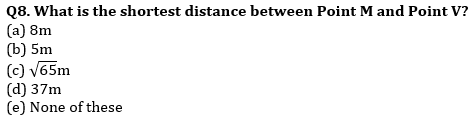Directions (9-10): Study the following information carefully to answer the given questions.
A person starts walking from point P in the east direction and walks 8m, then takes a right turn and walks 5m to reached at point Q. From point Q he takes two consecutive left turns and walks 10m and 3m respectively to reach at point R, then finally takes a left turn and walks 4m to reach at point S.

Q9. What is the shortest distance between point S and Q?
(a) 10m
(b) √21m
(c) 16m
(d) √45m
(e) None of these

Q10. What is the direction of point R with respect to point P?
(a) East
(b) North
(c) North-West
(d) South-East
(e) None of these

Directions (11-14): Study the information carefully and answer the questions given below.
A person starts walking from A towards to reach B, which is 15m east to A. He then takes a right turn and walks 20 m to reach point C. From C, he takes left turn and walks15m to point D, then again a left turn and walks 10m to point E. From E, he takes a left turn and walks 20m to reach point F. He then takes a right turn and walks 5m to reach G and finally a left turn to reach point H, which is 10 m away from G.

Q11. If J is 5m north to G, then what is the distance between A and J?
(a) 5m
(b) 10m
(c) 15m
(d) 20m
(e) none of these

Q12. Four of the following five belongs to a group find the one that does not belong to that group?
(a) A, G
(b) F, C
(c) B, H
(d) E, C
(e) D, E

Q13. What is the shortest distance between point A and H?
(a) 5m
(b) 10m
(c) 15m
(d) 20m
(e) none of these

Q14. Which of the following is the nearest point to X, which is the mid-point of BC?
(a) E
(b) F
(c) H
(d) B
(e) H

Q15. From my house, I starts to walk in east direction and covers a distance of 14 meters then takes a move to south and covers a distance of 20 meters and again takes a move to west and covers a distance of 9 meters and finally I moves to north and covers a distance of 8 meters and reaches to my friend’s house. My friend’s house is in which direction from my house?
(a) South
(b) East
(c) South-west
(d) South-east
(e) North-west

Solutions